## Register Now

Lorem ipsum dolor sit amet, consectetur adipiscing elit.Morbi adipiscing gravdio, sit amet suscipit risus ultrices eu.Fusce viverra neque at purus laoreet consequa.Vivamus vulputate posuere nisl quis consequat.

Digital to Analog Converter (DAC) is an integrated circuit that converts digital signal to analog voltage/current which is necessary for further Analog Signal Processing. This post will discuss in detail about what is Digital to Analog Converter (DAC), it’s types, how it works, applications, advantages and disadvantages.

## What is Digital to Analog Converter (DAC)

Digital to Analog Converter (DAC) basically converts digital code that represents digital value to analog current or voltage.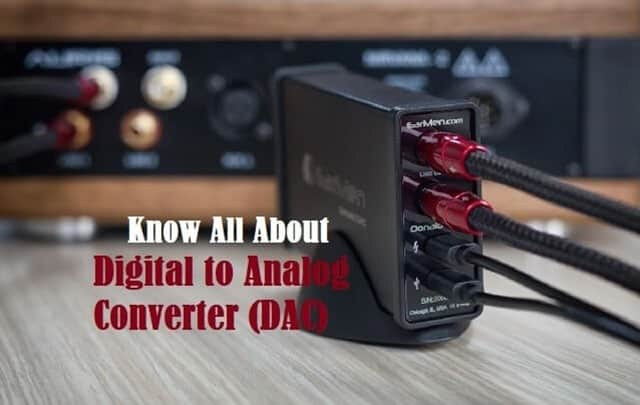Fig. 1 – Introduction to Digital to Analog Converter

In any communication system, the analog signal which is in the form of physical variables is converted to digital value by ADC. This digital signal is converted back to Analog signal for further processing i.e. Analog signal is necessary to drive Motors, temperature controller etc.

Fig.2 shows a block diagram of DAC circuit which shows n-bit digital inputs converted to Analog Signal. The digital inputs are d0, d1, dn-2, dn-1 and Va is the output Analog Voltage.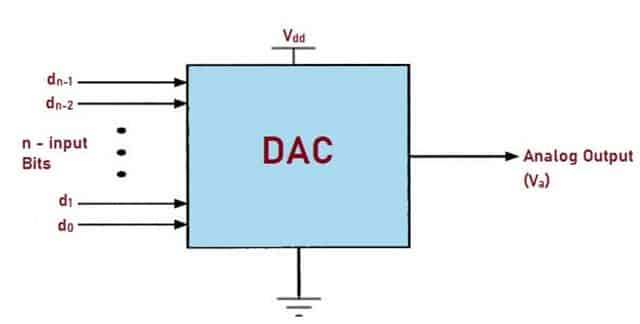Fig. 2 – Block Diagram of Digital to Analog Converter (DAC)

## Types of Digital to Analog Converter (DAC)

There are basically four types of Digital to Analog Converter circuits namely:

• Binary Weighted Resistor D/A Converter Circuit
• Segmented DAC
• Delta-Sigma DAC

### Binary Weighted Resistor D/A Converter Circuit

In this type of Converter, every digital input bit that needs to be converted, requires one resistor or current source. These resistors are connected across the inputs and the summing point. The output is generated through this Summing Amplifier Circuit. Fig. 3 below shows a typical Binary Weighted Resistor Converter Circuit which consists of an Op-Amp, four resistors which are connected at the input terminal of Op-amp along with the Feedback Resistor.

These Resistors at the input terminal are called as Variable Resistors. Here, A, B, C, D are the digital inputs where ‘D’ is at MSB and ‘A’ is at LSB. and V is the output Analog Voltage. The output of the Summing Amplifier circuit is given by the equation:The output voltage is obtained by substituting different values of inputs in the equation as shown in the table below:Fig. 3 – Binary Weighted Resistor Circuit

This type of Converter has only two values of Resistors, R and 2R. The conversion speed reduces in this type of DAC due to parasitic capacitance. It is the simplest type of DAC where the switch between ground and inverting input of the Op-amp is controlled by the input bit.

Fig.4 shows Binary Ladder or R–2R Ladder DAC. To understand its working, let us consider only the Resistors in the network, omitting Op-amp and assuming the input to be DCBA = 1000. Now the output for the reduced network is given by-Similarly, with the change in the input bits, the voltage will be V/4, V/2, V/16…… etc. Now, adding the Op-amp to the circuit, V4 becomes the input to the non-inverting Amplifier whose Gain is defined by the equation:Hence the output voltage of R–2R Ladder DAC is given by the equation: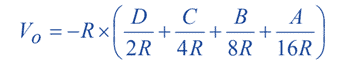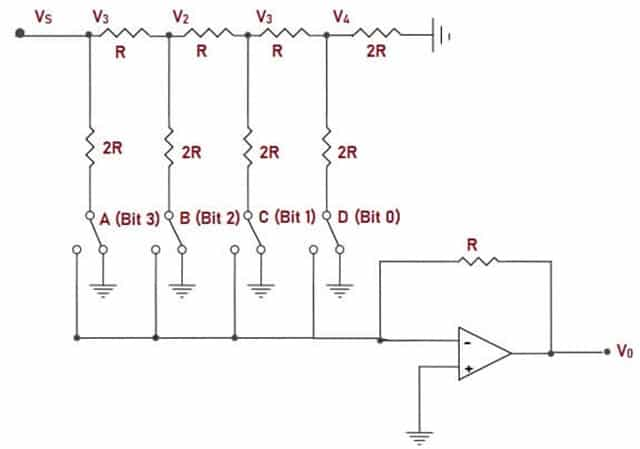### Segmented DAC

Segmented DAC’s are designed according to the specifications based on performance. In such cases no architecture is ideal and hence two or more DAC’s are combined and designed. Binary Weighted and Thermometer-coded DAC are combined. The input binary code is separated into 2 segments.

Thermometer-coding is used for MSB and Binary-weighted structure is applied for LSB. This is done to shrink the size of the chip. The size of the encoder grows exponentially with the increase in input bits, and more switches and interconnection is required.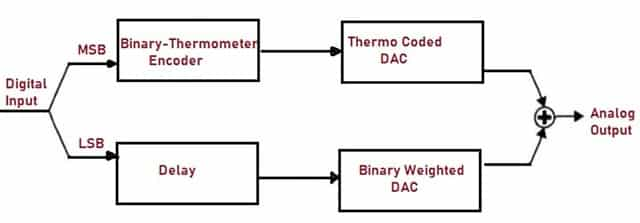Fig. 5 – Segmented DAC

### Delta-Sigma DAC

It is the fastest and highest precision DAC. Fig. 6 shows Delta-Sigma DAC which consists of different blocks namely:

• Interpolation Filter
• Delta-Sigma Modulator
• 1-Bit DAC
• Analog Output Filter

#### Interpolation Filter

It increases the sample rate and reduces the time and thus, sampling frequency is increased by four times. The data from the Filter is the input to the Modulator block.

#### Delta-Sigma Modulator

Delta-Sigma Modulator acts as a High-Pass Filter to the quantization noise and Low-Pass Filter to the signal. It converts data into high speed bit-stream.

#### 1-Bit DAC

Digital samples are converted back into Analog form for further amplification. Each bit of the sample is converted serially.

#### Analog Output Filter

The output from DAC is filtered out to produce Analog signal.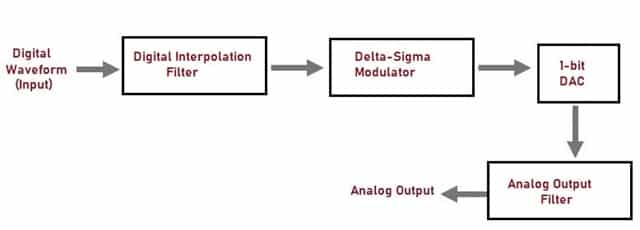Fig. 6 – Delta-Sigma Digital to Analog Converter

## How does Digital to Analog Converter (DAC) Work

To understand the working principle of Digital to Analog Converter, let us imagine a User on a computer recording an audio through a microphone. Here the speech or the audio signal is a physical variable and this signal has to be converted in to digital format. This is done by ADC (Analog to Digital Converter). This bit stream is processed by Digital Signal Processing techniques.

If the recorded audio has to be played through speakers, then the Digital signal has to be converted back to Analog signal with the help of Digital to Analog Converter.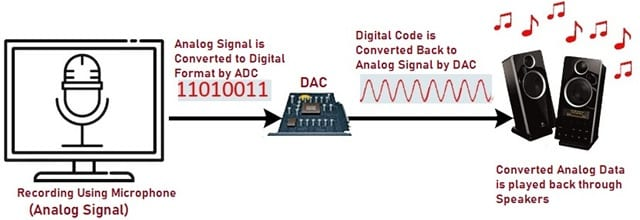Fig. 7 – Working Principle of DAC

## Applications of Digital to Analog Converter (DAC)

The applications of Digital to Analog Converter include:

• DAC’s are used in Digital Signal Processing.
• They are also used in digital power supplies for Micro-controller.
• DAC’s are used in digital potentiometers.
• They are used in all digital data acquisition systems.

## Advantages of Digital to Analog Converter (DAC)

The advantages of Digital to Analog Converter are:

• Weighted Resistor Circuit is the fastest converting circuit compared to other methods.
• High resolution and accuracy can be achieved.
• DAC’s are simple to implement.

## Disadvantages of Digital to Analog Converter (DAC)

```Also Read: